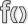# Plot.SetPlotType (Civ5 API)

Jump to: navigation, search

This page is a part of the Lua and UI Reference (Civ5).This function is a member of Plot. This is an instance method, invoke it with a colon.

# Usage

`void Plot:SetPlotType(PlotType terrain, bool recalculateAreas = nil, bool rebuildGraphics) = nil)`

Parameters

 terrain: No description available. recalculateAreas: No description available. rebuildGraphics): No description available.

# Source code samples

Redundant occurences have been removed.

Amazon_XP.lua (G&K)

`DLC/Expansion/Maps/Amazon_XP.lua`
 `0344` `plot:SetPlotType(PlotTypes.PLOT_HILLS, false, true); -- These flags are for recalc of areas and rebuild of graphics. Instead of recalc over and over, do recalc at end of loop.`

Arborea.lua (G&K)

`DLC/Expansion/Maps/Arborea.lua`
 `0611` `plot:SetPlotType(PlotTypes.PLOT_HILLS, false, false);`

AssignStartingPlots.lua (G&K)

`DLC/Expansion/Gameplay/Lua/AssignStartingPlots.lua`
 `2856` `forcePlot:SetPlotType(PlotTypes.PLOT_LAND, false, true);` `3577` `plot:SetPlotType(PlotTypes.PLOT_HILLS, false, true);` `5946` `plot:SetPlotType(PlotTypes.PLOT_MOUNTAIN, false, false);` `5950` `plot:SetPlotType(PlotTypes.PLOT_LAND, false, false);` `9534` `plot:SetPlotType(PlotTypes.PLOT_LAND, false, true)` `9535` `end`

Europe.lua (G&K)

`DLC/Expansion/Maps/Europe.lua`
 `1132` `adjPlot:SetPlotType(PlotTypes.PLOT_LAND, false, false);`

MapGenerator.lua (G&K)

`DLC/Expansion/Gameplay/Lua/MapGenerator.lua`
 `0173` `plot:SetPlotType(plotTypes[i + 1], false, false);` `0567` `plot:SetPlotType(PlotTypes.PLOT_OCEAN);`

NaturalWondersCustomMethods.lua (G&K)

`DLC/Expansion/Gameplay/Lua/NaturalWondersCustomMethods.lua`
 `0144` `plot:SetPlotType(PlotTypes.PLOT_OCEAN, false, false);` `0156` `SEPlot:SetPlotType(PlotTypes.PLOT_OCEAN, false, false);` `0219` `adjPlot:SetPlotType(PlotTypes.PLOT_MOUNTAIN, false, false);`

Skirmish.lua

`Maps/Skirmish.lua`
 `0255` `plot:SetPlotType(PlotTypes.PLOT_HILLS, false, false)` `0256` `break`

The initial version of this page was created by the Civ5 API Bot, see the Civ5 API Reference FAQ. Some of the texts come from the 2kgames' wiki and most of code samples are copyrighted to Firaxis.
Functions' signatures were either copied from the 2kgames' wiki, or infered from the Lua source files and the binaries. Errors are possible.
Contributors may find help in the Contributors guide to the Civ5 API.